May 2019

Volume 34 Number 5

# Using Survival Analysis for Predictive Maintenance

By Zvi Topol | May 2019

Some years ago, I introduced the basics of survival analysis and described how to implement a non-parametric algorithm called Kaplan-Meier in C# (msdn.com/magazine/dn630650). Now, I’m going to take another look at survival analysis, in particular at two more advanced methodologies that are readily available on two popular machine learning platforms, Spark Machine Learning Library (MLLib) and h2o.ai, which are both supported by Azure HDInsight. I’ll use a predictive maintenance use case as the ongoing example.

## Predictive Maintenance for the Industrial Internet of Things

The main idea behind the Industrial Internet of Things (IIoT) is to connect computers, devices, sensors, and industrial equipment and applications within an organization and to continually collect data, such as system errors and machine telemetry, from all of these with the aim of analyzing and acting on this data in order to optimize operational efficiencies.

The goal of predictive maintenance is to accurately predict when a machine or any of its components will fail. If you can do this, you can perform maintenance just before such failure is predicted to occur. This is more efficient than not performing any maintenance until a failure occurs, in which case the machine or component will be unavailable until the failure is fixed, if indeed it’s reparable. Such unplanned downtime is likely to be very costly.

Predictive maintenance is also more effective than performing preventive maintenance at frequent intervals, which could also be costlier because unnecessary maintenance may be applied.

The example and the data I’ll use are an adapted version of the example at bit.ly/2J4WnbN. The example includes 100 manufacturing machines, with no interdependencies among the machines. Each machine is one of four possible models.

The data for the machines includes a history of failures, maintenance operations and sensor telemetry, as well as information about the model and age (in years) of the machines. This data is available in .csv files downloadable from the resource mentioned earlier. I’ll also provide a transformed data file (comp1_df.csv) that’s “survival analysis-ready” and will explain how to perform the transformations later on.

Each machine in the original example has four different components, but I’m going to focus only on one component. The component can either be maintained proactively prior to a failure, or maintained after failure to repair it.

## Survival Analysis

In my previous article about survival analysis, I introduced important basic concepts that I’ll use and extend in this article. I encourage you to read that article to familiarize yourself with these concepts, including the survival and hazard functions, censoring and the non-parametric Kaplan-Meier (KM) estimator.

In this article, I’ll show how to extend the concept of the KM estimator to include covariates or variables (also known as features) that can have effects on survival, or, in this case, on machine components’ failure. In the example, I’ll use machine model, machine age and machine telemetry as covariates and use survival regression models to estimate the effects of such covariates on machine failure.

The notion of estimating the effects of covariates on a target variable, in this case time to failure, hazard rate, or survival probabilities, isn’t unique to survival analysis and is the basis for regression models in general.

When building statistical models, you see covariates of three primary data types: categorical, ordinal and continuous. Categorical data types are those types that fall into a few discrete categories. Here, a machine model is a categorical data type—there are four different machine models. Ordinal data types are categorical data types that have some meaningful order. For example, ratings of movies from one to 10, where 10 is the most entertaining and one the least. Finally, continuous data types are those that represent continuous numbers. Those would be the machine telemetry readings here, which are continuous numbers sampled at certain times (in this case, hourly).

After identifying the data types and the methodology to be used, you should encode the various data types into covariates. Typically, for regression models, continuous variables are naturally encoded as continuous covariates, while categorical data types will require some form of encoding. A popular option for such encoding, which I’ll use in this article, is where, for categorical data types with N categories, N-1 covariates are created, and a category i is represented by setting its specific covariate to value one and all others to zero. The Nth category is represented by setting all covariates to zero. This is typically a good fit for regression models with an explicitly defined baseline, where all covariates can be equal to zero. This is also the format that the R programming language uses to encode categorical variables or factors.

This encoding for categoricals has a straightforward interpretation for what it means for some or all covariates to be set to zero. However, for continuous data types, setting a certain covariate to zero may not always be meaningful. For example, if a covariate represents machine height or width, setting that covariate to zero would be meaningless, because there are no such machines in reality.

One way around this problem is to use mean centered continuous covariates, where for a given covariate, its mean over the training dataset is subtracted from its value. Then, when you set that transformed covariate to zero, it’s equivalent to setting the original covariate to its mean value. This technique is called “mean centering” and I’ll use it here for the machine age and telemetry covariates.

It’s important to remember, that following this transformation, you should always use mean centered covariates as an input to the model. This is also the case when applying the regression model to a new test dataset.

Once the data values are encoded as covariates, survival regression models then take those covariates and a certain form of survival target variables (which I’ll talk about soon) and specify a model that ties the effects of such covariates on survival/time-to-event.

## Transformation of the Data to Survival Format and Feature Engineering

In order to work with the survival regression models that I’ll describe, your data needs to have at least two fields: the time stamp of the event of interest (here, machine failure) and a Boolean field indicating whether censoring occurred. (Here, censoring describes a situation in which no failure occurred at or before a specified time. In my example, maintenance happening in a preventive manner, rather than as a response to failure, is considered to be censoring.

The survival regression models I’ll discuss have different assumptions made to simplify their mathematical derivation. Some of these assumptions may not hold here, but it’s still useful to apply survival modeling to this example.

The survival analysis literature is very rich and many advanced survival regression models and techniques have been developed to address and relax some of these assumptions. You can read more about such models and techniques in the book, “The Statistical Analysis of Failure Time Data” by Kalbfleisch and Prentice (Wiley-Interscience, 2002), at bit.ly/2TACdLR.

I’ll make the assumption that each maintenance operation performed on a machine component completely resets that component and can therefore be treated independently. It’s then possible to use survival regression on two types of intervals (depicted in Figure 1):

• The interval between a failure and the preceding maintenance operation (time to event).
• The interval between subsequent maintenance operations (censoring).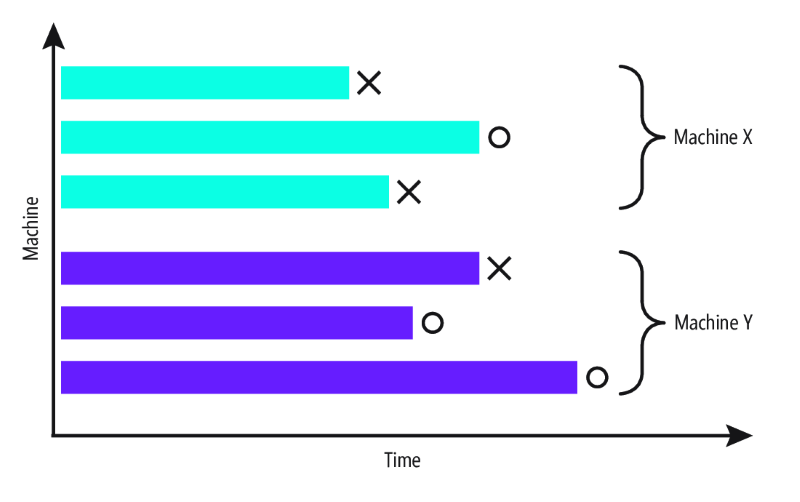Figure 1 Survival Representation of Machine Failures

Each interval in Figure 1 starts with a maintenance operation. The first type of interval ends with X, denoting a failure, while the second type ends with O, denoting another maintenance operation prior to a failure (this is essentially a proactive maintenance operation), which in this case means a censored observation.

Therefore, the original data needs to be transformed into this format with the two required fields. The “time_to_event” field represents the time in hours until either failure or the next maintenance occurs. The “event” field is set to one for a failure and to zero for a maintenance operation before failure.

It’s frequently desirable to perform additional transformations on the covariates, which is often called “feature engineering.” The purpose of this process is to generate covariates with better predictive power. For example, you can create another covariate that will calculate the mean of the pressure in the 10 hours prior to failure. There are many different options for functions and possible time windows to create such covariates, and there are a few tools you can use to help automate this process, such as the open source Python package tsfresh (tsfresh.readthedocs.io/en/latest).

Now I’m going to discuss the two survival regression models: the Cox proportional hazard model (or Cox PH model) available in h2o.ai and the Weibull Accelerated Failure Time model available in Spark MLLib.

## Cox Proportional Hazards Regression

Recall that a hazard function determines the event rate at time t for objects or individuals that are alive at time t. For the predictive maintenance example, it can be described as the probability of failing in the next hour, for a given time t and for all the machines where component 1 failure hasn’t occurred since their last maintenance. Higher hazard rates imply higher risk of experiencing failure. The Cox PH regression estimates the effects of covariates on the hazard rate as specified by the following model: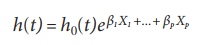Here, h(t) is the hazard function at time t, h0(t) is the baseline hazard at time t, the Xi variables are the different covariates and the corresponding betas are coefficients corresponding to the covariates (more on that a bit later). The baseline hazard is the hazard when all covariates are equal to zero. Note that this is closely related to the intercept in other regression models, such as linear or logistic regression.

According to this model, there’s no direct relationship between the covariates and the survival time. This model is called semi-parametric because the hazard rate at time t is a function of both a baseline hazard rate that’s estimated from the data and doesn’t have a parametric closed form and a multiplicative component that’s parameterized.

The reason this model is called a proportional hazard model is because it allows you to compare the ratio of two hazard functions. In this case, given an estimated model, the ratio between two different data points is:The baseline hazard rate cancels out and the resulting ratio between the hazards is only a function of coefficients and covariates and again doesn’t depend on time. This is closely related to logistic regression where the log of the odds is estimated. Also, the Cox PH regression model doesn’t directly specify the survival function, and the information it provides focuses on the ratio or proportion of hazard functions. Therefore, it’s primarily used to understand the effects of covariates on survivability, rather than to directly estimate the survival function.

With the Cox PH model specified, the coefficients and the non-parametric baseline hazard can be estimated using various techniques. One popular technique is partial maximum likelihood estimation (also used in h2o.ai).

The following code snippet is an R script that runs an estimation of the Cox PH model using h2o.ai on the mean centered covariates (machine telemetry and age) and the categorical covariate machine model:

``````library(h2o)
localH2O <- h2o.init()
inputFileName<-'comp1_df.csv'
df.hex <- as.h2o(df, key = "df.hex")
model <- h2o.coxph(x = c("age_mean_centered", "model","volt_mean_centered",
"rotate_mean_centered","pressure_mean_centered", "vibration_mean_centered"),
event_column = "event", stop_column ="time_to_event" ,training_frame = df.hex)
summary(model)
``````

At the time of this writing, the Cox PH model in h2o.ai isn’t available to use from Python, so R code is provided. Installation instructions are available at bit.ly/2z2QweL, or, for h2o.ai with Azure HDInsight, at bit.ly/2J7nXp6.

Running the code snippet generates the output shown in Figure 2.

Figure 2 Output for the Cox PH Regression

``````Surv(time_to_event, event) ~ model + volt_mean_centered + rotate_mean_centered +
pressure_mean_centered + vibration_mean_centered + age_mean_centered
n= 709, number of events= 192
coef      exp(coef) se(coef)    z      Pr(>|z|)
model.model2                  -0.066955  0.935237  0.257424 -0.260    0.795
model.model3                  -0.021837  0.978400  0.215614 -0.101    0.919
model.model4                   0.308878  1.361896  0.227469  1.358    0.174
volt_mean_centered             0.031903  1.032418  0.003990  7.995    1.33e-15 ***
rotate_mean_centered           0.001632  1.001633  0.001362  1.199    0.231
pressure_mean_centered        -0.008164  0.991869  0.005768 -1.415    0.157
vibration_mean_centered        0.018220  1.018387  0.013866  1.314    0.189
age_mean_centered              0.004804  1.004815  0.013293  0.361    0.718
---
Signif. codes:  0 ‘***’ 0.001 ‘**’ 0.01 ‘*’ 0.05 ‘.’ 0.1 ‘ ’ 1
exp(coef) exp(-coef) lower .95 upper .95
model.model2                      0.9352     1.0692    0.5647     1.549
model.model3                      0.9784     1.0221    0.6412     1.493
model.model4                      1.3619     0.7343    0.8720     2.127
volt_mean_centered                1.0324     0.9686    1.0244     1.041
rotate_mean_centered              1.0016     0.9984    0.9990     1.004
pressure_mean_centered            0.9919     1.0082    0.9807     1.003
vibration_mean_centered           1.0184     0.9819    0.9911     1.046
age_mean_centered                 1.0048     0.9952    0.9790     1.031
Rsquare= 0.094   (max possible= 0.941 )
Likelihood ratio test= 70.1  on 8 df,   p=4.69e-12
Wald test            = 70.19  on 8 df,   p=4.514e-12
``````

The first important thing to note is the estimated coefficients of the covariates. The machine model covariate is encoded as a categorical data type. The baseline for this category is model1, which is represented by setting the three covariates encoding the other three machine models (model.model2, model.model3 and model.model4) to zero. Each covariate gets its own coefficient. Understanding how to interpret the coefficients is important.

If you apply the exponential function to the coefficients for the machine model covariates (exp(coeff) in the output), you see that model.model2 has a value of 0.9352, while model.model4 has a value of 1.3619. This means that machines of model2 have a hazard rate that’s 6.5 percent lower than the hazard rate of the baseline machine model (model 1), and that machines of model.model4 have a considerably higher hazard of 36.2 percent compared to machines of model.model1. In other words, machines of model.model4 have the highest risk of failure, while machines of model.model2 have the lowest risk of failure. Therefore, when prioritizing maintenance operations, the model of the machine should be an important factor to take into consideration.

All other covariates are mean centered continuous covariates. The interpretation of the coefficients affiliated with them is that now the hazard ratio is given by the exponential of the covariates around their means. Therefore, by increasing a covariate value by one unit (keeping all other covariates fixed), the hazard ratio increases (or decreases) by the exponential of the coefficient (in a similar way to that of the categorical variable). So, for example, by increasing the voltage by one unit, the risk for failure increases by 3.2 percent.

Another important point to mention here concerns model diagnostics techniques. Such techniques provide a basis to understand whether the model considered (in this case, the Cox PH model) is appropriate. Here, the Rsquare value (a value between zero and one, the higher the better) is relatively low (0.094) and most of the z-scores of the coefficients don’t indicate that the coefficients are statistically significant (there isn’t enough evidence to support that they’re different from zero). Both of these indicators lead to the conclusion that there’s room for improvement, for example through feature engineering. There are also other statistical tests that are specific to the Cox PH model that should be conducted. You can consult the survival analysis literature I mentioned earlier for more details.

## The Accelerated Failure Time Model

The survival regression model in Spark MLLib is the Accelerated Failure Time (AFT) model. This model directly specifies a survival function from a certain theoretical math distribution (Weibull) and has the accelerated failure time property.

The AFT model is defined as follows. Assume an object is characterized by using the (linear) covariates and coefficients:Also assume that the object has a parametric survival function s(t) and, denoted by s0(t), the survival function of a baseline object (with all covariates set to zero). The AFT model defines the relationship between s(t) and s0(t) as:From this definition you can see why the model is called Accelerated Failure Time model. It’s because the survival function includes an accelerator factor, which is the exponential function of the linear combinations of the covariates, which multiplies the survival time t.

This type of model is useful when there are certain covariates, such as age (in my dataset, machine age), that may cause monotonic acceleration or deceleration of survival/failure time.

The Weibull distribution is a generalization of the exponential distribution and is a continuous distribution popular in parametric survival models. There are a few variations on how to parameterize it. Here, I’ll use the following two-parameter Weibull distribution version for t>=0:(There are also versions with three parameters.) The two parameters of the distribution are the shape that’s determined by k and the scale that’s determined by lambda. A rough analogy is the way a bell-shaped distribution has a characteristic mean and standard deviation.

Recall that the relationship between the distribution density function f(t), the hazard function h(t) and the survival function s(t) is given by f(t) = h(t)s(t).

The following are the Weibull hazard and survival functions: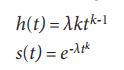Unlike the Cox PH model, both the survival and the hazard functions are fully specified and have parametric representations. Please refer to Figure 3 and Figure 4 for visualizations of the Weibull distribution and survival functions for different values of k and lambda.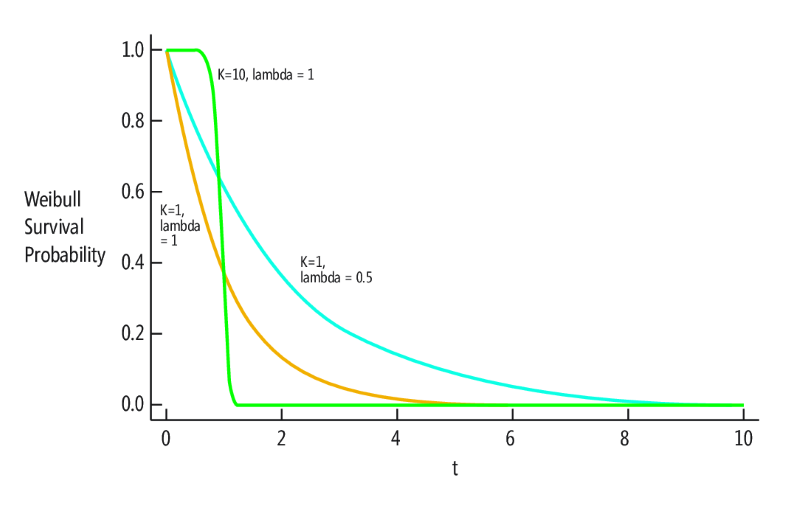Figure 3 Weibull Distribution Shape as a Function of Different Values of K and LambdaFigure 4 Weibull Survival Function Shape for Different Values of K and Lambda

Figure 5 illustrates the effects that AFT model covariates have on the shape of the Weibull survival function.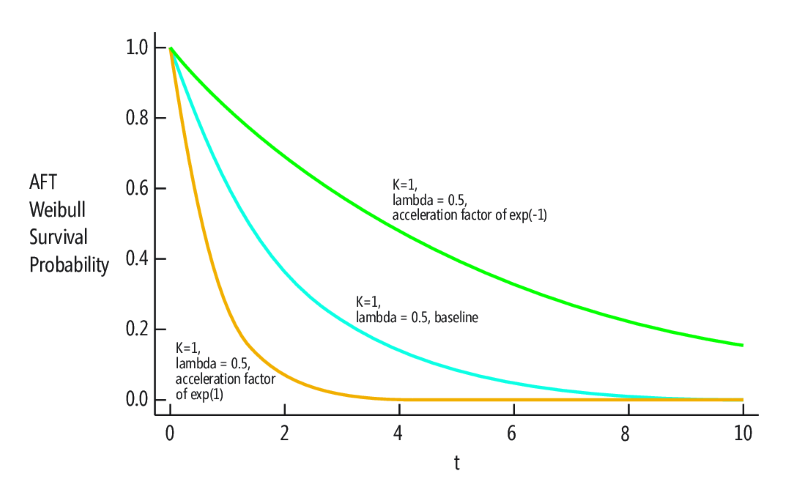Figure 5 Accelerated Failure Time for the Weibull Survival Probability Function

Estimation of the coefficients for the AFT Weibull model in Spark MLLib is done using the maximum likelihood estimation algorithm. You can learn more about how it’s done at bit.ly/2XSauom, and find the implementation code at bit.ly/2HtJw0v.

Unlike the estimation of the Cox PH model, where only the coefficients of the covariates are reported (along with some diagnostics), the results obtained from estimating the Weibull AFT model report the coefficients of the covariates, as well as parameters specific for the Weibull distribution—an intercept and a scale parameter. I’ll show how to convert those to k and lambda in a bit.

The results for the Weibull AFT implementation in Spark MLLib match the results for the Weibull AFT implementation using the survreg function from the popular R library “survival” (more details are available at bit.ly/2XSxkw8).

You can run the following R script for the AFT Weibull model estimation (the code runs on a locally installed Spark MLLi, but you can also use Spark on HDInsight at bit.ly/2u2U5Qf):

``````library(survival)
library(SparkR, lib.loc = c(file.path(Sys.getenv("SPARK_HOME"), "R", "lib")))
sparkR.session(master = "local[*]")
inputFileName<-'comp1_df.csv'
aftMachineDF<-suppressWarnings(createDataFrame(df))
aftMachineModel <- spark.survreg(aftMachineDF,Surv(time_to_event, event) ~ model +
age_mean_centered +  volt_mean_centered + rotate_mean_centered +
pressure_mean_centered + vibration_mean_centered)
summary(aftMachineModel)
``````

The script generates only the estimated coefficients without additional information. It’s possible to get such information by running survreg (because results match):

``````library(survival)
machineModel<-survreg(Surv(time_to_event, event) ~ model + age_mean_centered +
volt_mean_centered+rotate_mean_centered + pressure_mean_centered +
vibration_mean_centered, df, dist='weibull')
summary(machineModel)
``````

In this case, the R script generates the more elaborate output shown in Figure 6.

Figure 6 Output for the Weibull AFT Regression

``````survreg(formula = Surv(time_to_event, event) ~ model + age_mean_centered +
volt_mean_centered + rotate_mean_centered + pressure_mean_centered +
vibration_mean_centered, data = df, dist = "weibull")
Value        Std. Error    z            p
(Intercept)                 8.172991       0.119133    68.6040     0.00e+00
modelmodel2                 0.040289       0.154668    0.2605      7.94e-01
modelmodel3                 0.027225       0.129629    0.2100      8.34e-01
modelmodel4                  -0.163865      0.136382   -1.2015     2.30e-01
age_mean_centered           -0.000753      0.007960    -0.0946     9.25e-01
volt_mean_centered           -0.019731      0.002583   -7.6391     2.19e-14
rotate_mean_centered         -0.000767      0.000821   -0.9334     3.51e-01
pressure_mean_centered       0.005173       0.003496     .4795     1.39e-01
vibration_mean_centered      -0.008214      0.008391   -0.9789     3.28e-01
Log(scale)                   -0.508060      0.051963   -9.7773     1.41e-22
Scale= 0.602
Weibull distribution
Loglik(model)= -1710.3   Loglik(intercept only)= -1747.2
Chisq= 73.73 on 8 degrees of freedom, p= 8.9e-13
Number of Newton-Raphson Iterations: 8
n= 709
``````

Before moving on to describe the output, I should mention that the Weibull parameterization in Spark MLLib and in survreg is a bit different than the parameterization I discussed.

A transformation is required and can be done as follows. Denote the parameters reported—intercept by m and scale by s—then k = 1/s, lambda = exp(-m/s) and each coefficient should be multiplied by (-1/s). There’s an R package called SurvRegCensCov that can do this conversion automatically, using ConvertWeibull on the model that survreg estimated:

``````\$vars
Estimate        SE
lambda                                    1.260459e-06   8.642772e-07
gamma                                     1.662064e+00   8.636644e-02
modelmodel2                               -6.696297e-02  2.569595e-01
modelmodel3                               -4.524990e-02  2.155000e-01
modelmodel4                               2.723541e-01   2.268785e-01
age_mean_centered                         1.251958e-03   1.322780e-02
volt_mean_centered                        3.279500e-02   3.947495e-03
rotate_mean_centered                      1.274045e-03   1.365339e-03
pressure_mean_centered                    -8.598142e-03  5.807130e-03
vibration_mean_centered                   .365213e-02    1.391255e-02
``````

Here, gamma is equal to k from the previous Weibull parameterization. (For more information on SurvRegCensCov, see bit.ly/2CgcSMg.)

Given the estimated parameters, unlike with the Cox PH model, it’s now possible to directly obtain the survival function (it’s the Weibull AFT survival function) and use it to predict survival probabilities for any covariates. Assuming the first point in the dataset is a new data point, you can run the following:

``````predict(machineModel, newdata=df[1,], type='quantile')
``````

This yields the time to event (in hours) for the quantiles 0.1 and 0.9 (the defaults), like so:

``````807.967 5168.231
``````

This means that given the covariates of the first data point (listed here), the probability of failure is 10 percent at or just before 807.967 hours following a maintenance operation, and the probability of failure is 90 percent at or just before 5168.231 hours following the maintenance operation:

``````model       age           volt_mean_centered                 rotate_mean_centered
model3       18                  3.322762                          51.8113
pressure_mean_centered     vibration_mean_centered             age_mean_centered
10.10773                         11.4267                           6.488011
``````

You can also use parameter “p” to get the survival time for any quantiles between zero and one; for example, adding the parameter “p=0.5” will give the median failure time, which, for the first data point, is 2509.814 hours after a maintenance operation.

As with the Cox PH model estimation, the p column in the output of survreg provides information about the statistical significance of the coefficients estimated, though in this case the figures are better (lower p-values). There’s still room for feature engineering here as was described before for the Cox PH model.

It’s also important to perform model diagnostics here, as was the case in the Cox PH regression, to make sure that the Weibull AFT model is a good fit for the data, compared, for example, to other parametric models. While I won’t describe this process here, you can learn more about it by referring to the “Survival Analysis” book I mentioned earlier.

## Wrapping Up

I’ve presented the use of predictive maintenance for the IIoT as a motivating example for the adoption of two survival regression models that are available in h2o.ai and Spark MLLib. I showed how to model a machine failure predictive maintenance problem in the survival analysis framework by encoding variables as covar­iates and transforming the time series data to survival format. I also described the two survival models, the differences between them and how to apply them to the data. Finally, I talked briefly about interpretation of the results and model diagnostics. It’s important to note that I only scratched the surface of this fascinating and very rich topic, and I encourage you to explore more. A starting point for doing so is by referring to the literature I mentioned in the article.

Zvi Topol has been working as a data scientist in various industry verticals, including marketing analytics, media and entertainment, and Industrial Internet of Things. He has delivered and lead multiple machine learning and analytics projects, including natural language and voice interfaces, cognitive search, video analysis, recommender systems and marketing decision support systems. Topol is currently with MuyVentive LLC, an advanced analytics R&D company, and can be reached at zvi.topol@muyventive.com.

Thanks to the following Microsoft technical expert for reviewing this article: James McCaffrey